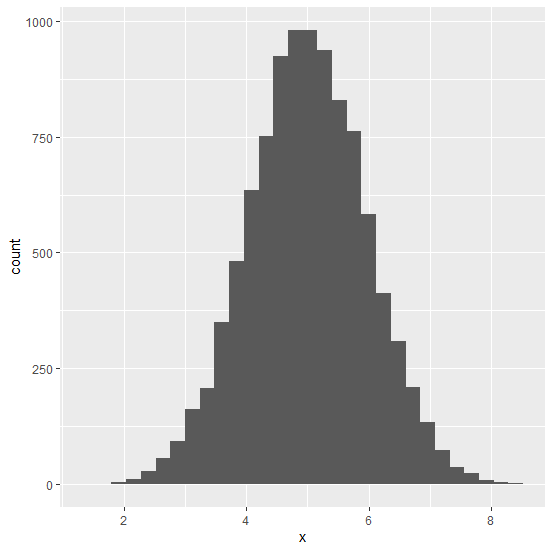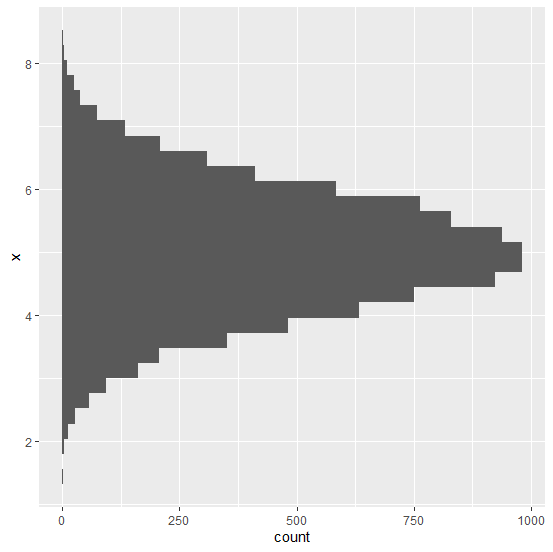# How to create horizontal histogram in R?

Generally, the histogram is a graph that is displayed in vertical form and it helps us to analyze the distribution of a variable, mainly to understand whether the distribution is normal or not. The horizontal histogram can be also created by using coord_flip function of ggplot2 package. Check out the below example to understand how it works.

## Example

Consider the below data frame.

Live Demo

> x<-rnorm(10000,5,0.97)
> df<-data.frame(x)
> head(df,20)

## Output

x
1 3.509446
2 5.075813
3 5.242884
4 5.236765
5 5.775746
6 5.331167
7 5.250956
8 5.925262
9 6.102322
10 4.045241
11 4.117635
12 4.137581
13 4.758140
14 5.311225
15 4.354592
16 4.021351
17 5.330966
18 5.376746
19 6.717059
20 5.206282

> library(ggplot2)
> ggplot(df,aes(x))+geom_histogram(bins=30)

## OutputCreating horizontal histogram of x.

> ggplot(df,aes(x))+geom_histogram(bins=30)+coord_flip()

## Output: